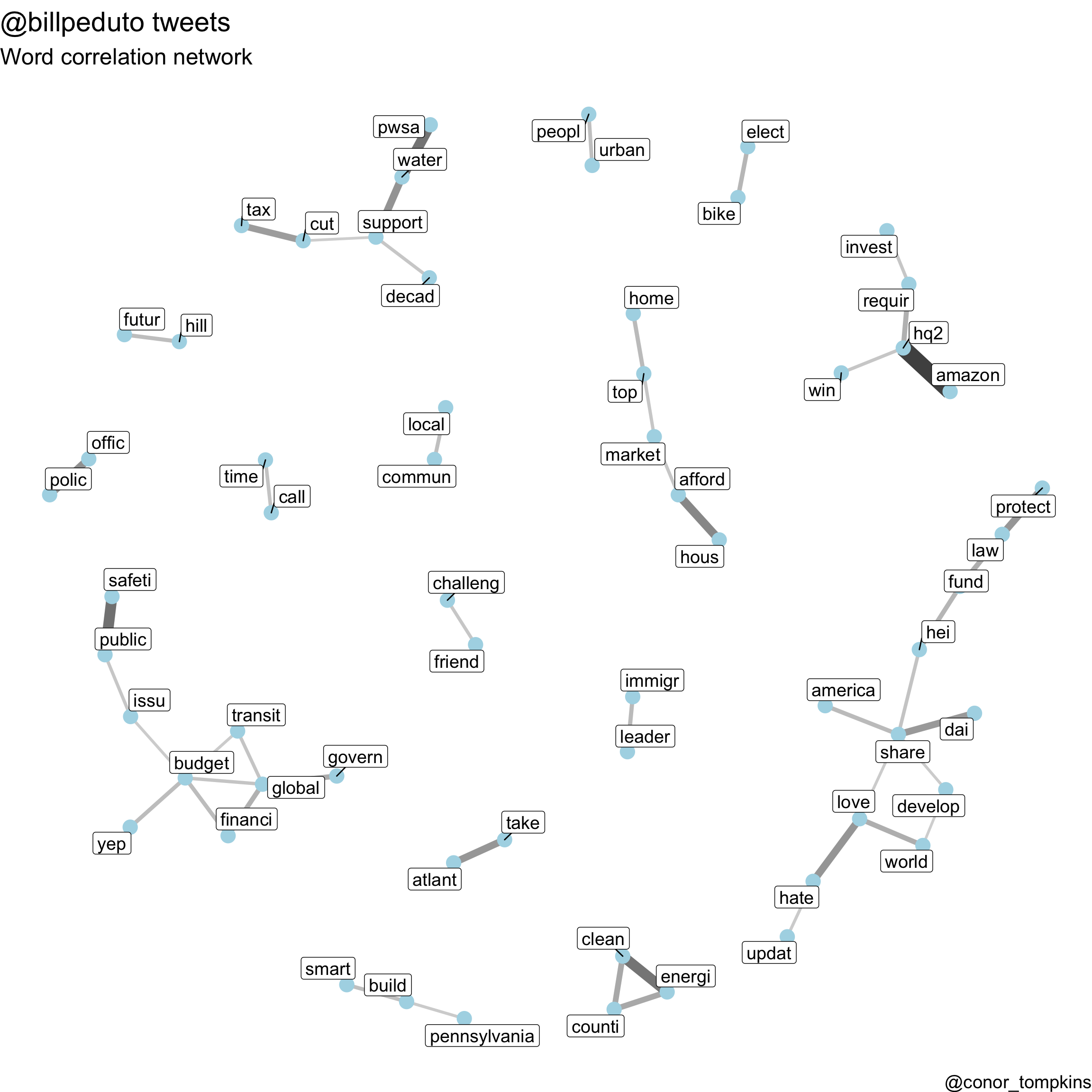# Mayor Bill Peduto Tweets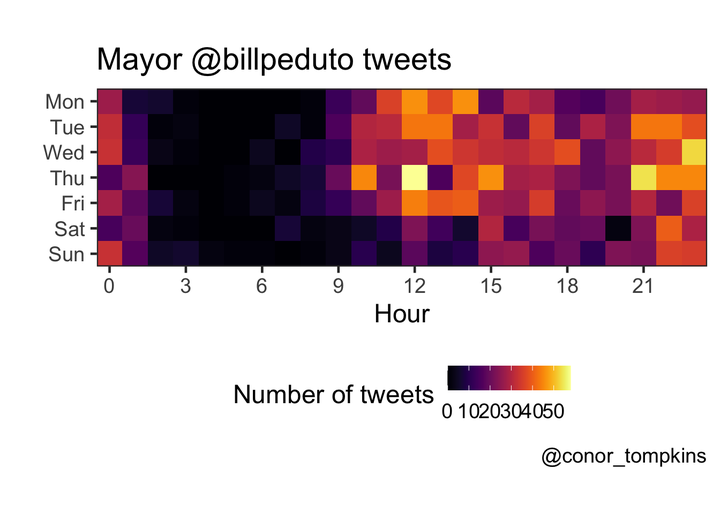Pittsburgh Mayor Bill Peduto uses Twitter to communicate with his constituents, express his political opinions, and comment about Pittsburgh sports. I will use various R packages (mainly the Tidyverse, Tidytext, and rtweet) to analyze how the Mayor uses Twitter.

Before getting started, we need to load the packages required for this analysis:

``````library(tidyverse)
library(tidytext)
library(ggraph)
library(igraph)
library(widyr)
library(SnowballC)
library(lubridate)
library(viridis)
library(scales)
library(knitr)
library(kableExtra)``````

``rtweet::tweets_bill <- get_timelines("BillPeduto", n = 3200)``

I have already downloaded the data, so I will load it from my GitHub repo. I will also do some data munging to make the data easier to work with.

``````url <- "https://raw.githubusercontent.com/conorotompkins/pittsburgh_twitter/master/data/df_billpeduto.csv"

mutate(created_at = with_tz(created_at, "US/Eastern"),
date = ymd(str_sub(created_at, 1, 10)),
year = year(date),
month = month(date, label = TRUE),
week = week(date),
wday = wday(date, label = TRUE),
hour = hour(created_at),
month_year = str_c(month, year, sep = "-"),
month_year = factor(month_year, levels = unique(month_year)),
wday = factor(wday, levels = c("Mon", "Tue", "Wed", "Thu", "Fri", "Sat", "Sun"))) -> df_bill

df_bill[1:5, 1:5] %>%
kable("html") %>%
kable_styling()``````
status_id created_at source is_quote is_retweet
x892785891055468548 2017-08-02 12:35:18 Twitter for iPhone FALSE TRUE
x892804872390795264 2017-08-02 13:50:43 Twitter for iPhone FALSE TRUE
x892820785823404032 2017-08-02 14:53:57 Twitter for iPhone FALSE TRUE
x892926762933506048 2017-08-02 21:55:04 Twitter for iPhone FALSE TRUE
x892953788679692288 2017-08-02 23:42:28 Twitter for iPhone FALSE FALSE

## Exploratory Analysis

This plot shows that the Mayor tweets about 15 times a day, though there is considerable daily variation.

``````df_bill %>%
count(date) %>%
ggplot(aes(date, n)) +
geom_jitter(alpha = .5) +
geom_smooth(size = 2) +
scale_x_date(date_breaks = "month",
date_labels = "%b-%Y") +
labs(title = title,
x = "",
y = "Number of tweets",
caption = caption)``````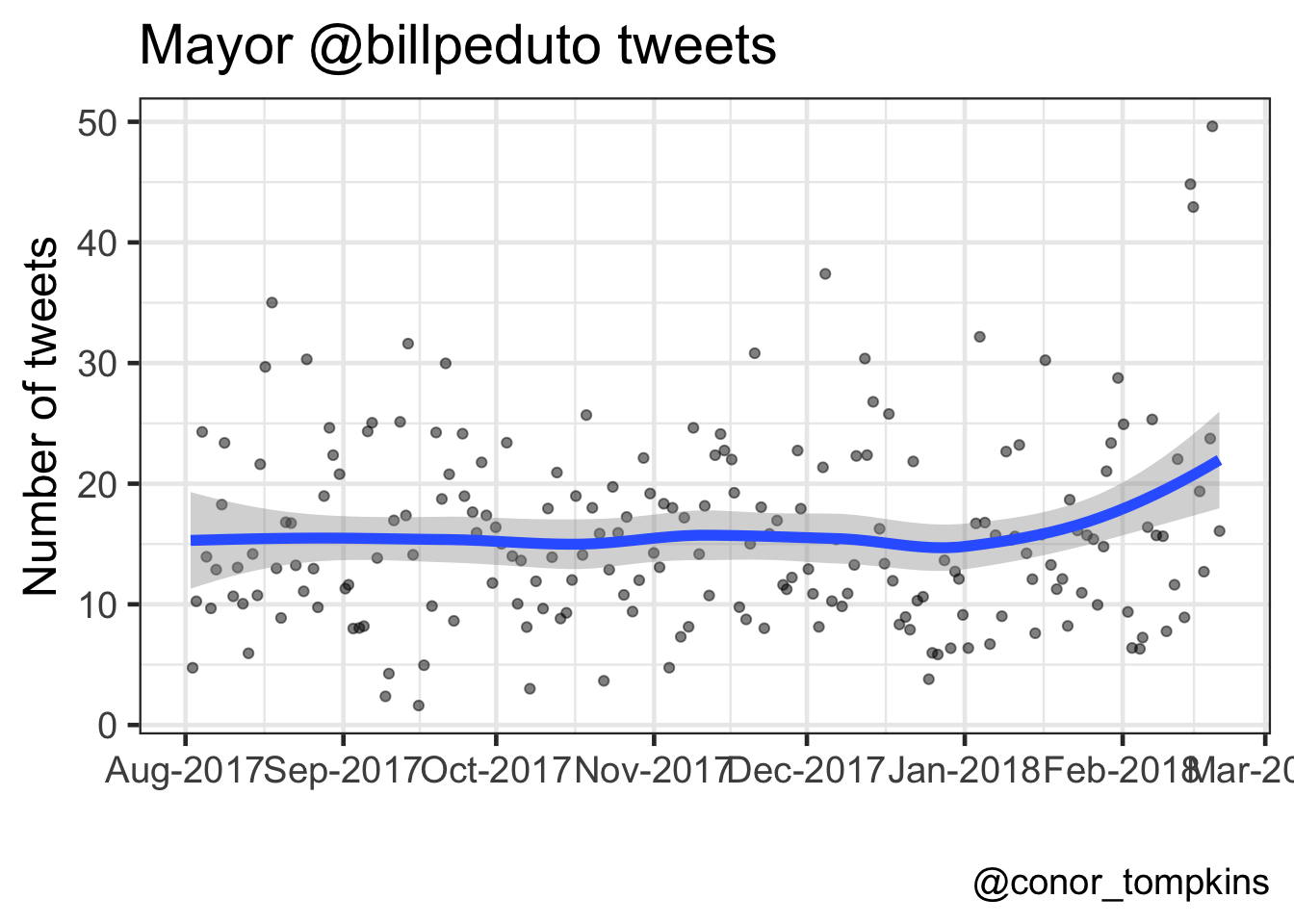The Mayor tweets the most on weekdays; Tuesday has the highest median number of tweets. The weekends see fewer tweets, and Saturday and Sunday are very similar.

``````df_bill %>%
count(wday, date) %>%
group_by(wday) %>%
mutate(median = median(n)) %>%
ggplot(aes(wday, n, color = wday)) +
geom_jitter(alpha = .3, width = .2) +
geom_point(aes(wday, median), size = 5) +
scale_color_viridis(name = "Day of the week", discrete = TRUE) +
labs(title = title,
subtitle = "One small dot = one day, large dot = median for weekday",
x = "",
y = "Number of tweets in a given day",
caption = caption) +
theme_bw(base_size = 18)``````The Mayor does most of his tweeting around lunchtime and in the late evening.

``````df_bill %>%
count(hour) %>%
ggplot(aes(hour, y = 1, fill = n)) +
geom_tile() +
coord_equal() +
scale_y_continuous(expand = c(0,0)) +
scale_x_continuous(expand = c(0,0),
breaks = seq(0, 23, by = 3)) +
scale_fill_viridis(name = "Number of tweets",
option = 3) +
guides(fill = guide_colorbar(title.position = "top")) +
labs(title = title,
x = "Hour",
y = "",
caption = caption) +
theme(axis.text.y = element_blank(),
axis.ticks.y = element_blank(),
legend.position = "bottom",
legend.text = element_text(size = 7))``````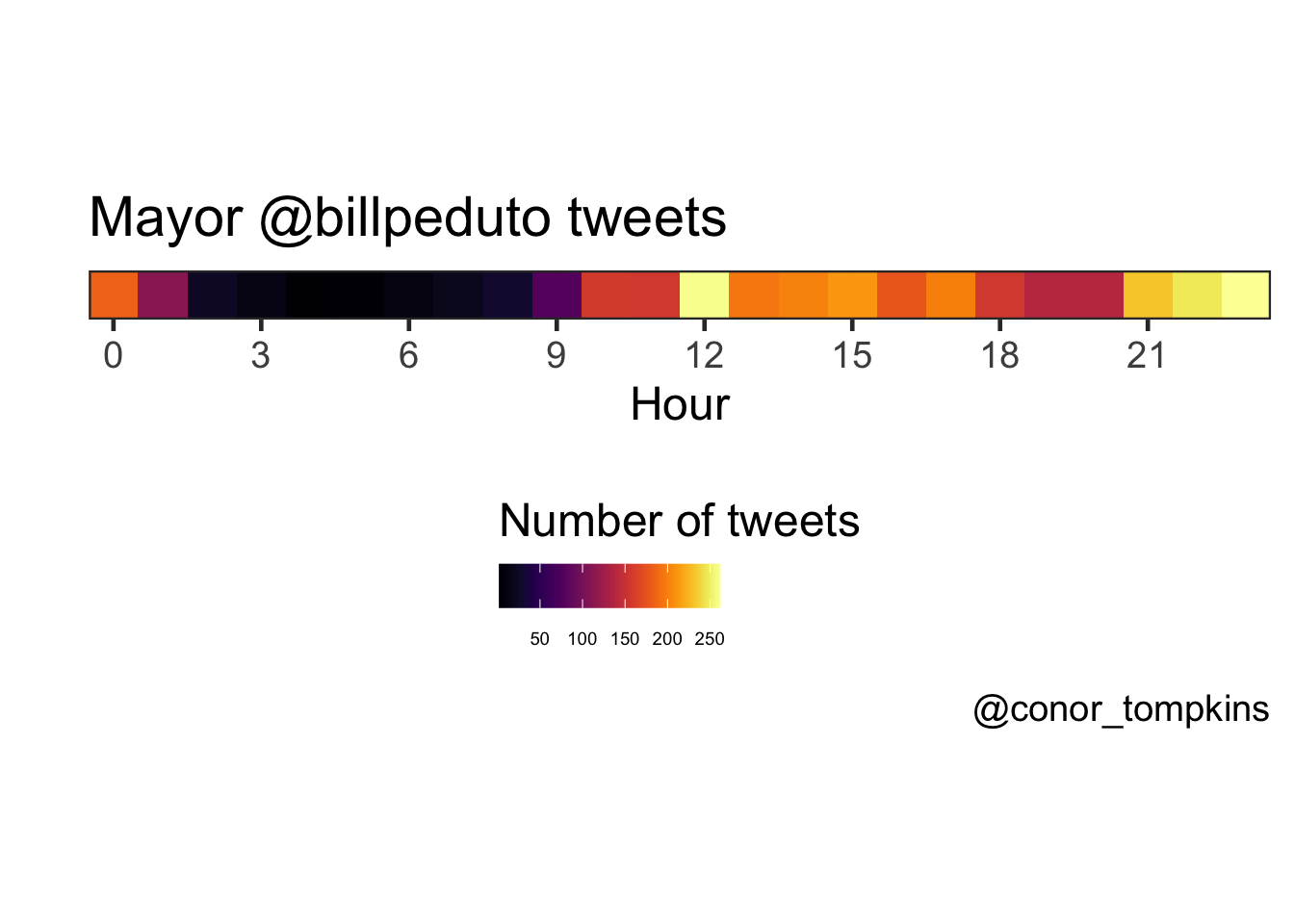This view combines the day of the week and hour variables, and confirms what we observed when looking at the variables independently.

``````df_bill %>%
mutate(wday = factor(wday, levels = rev(levels(df_bill\$wday)))) %>%
count(wday, hour) %>%
complete(wday, hour = 0:23) %>%
replace_na(list(n = 0)) %>%
ggplot(aes(hour, wday, fill = n)) +
geom_tile() +
coord_equal() +
scale_x_continuous(expand = c(0,0),
breaks = seq(0, 24, by = 3)) +
scale_y_discrete(expand = c(0,0)) +
scale_fill_viridis(option = 3) +
labs(title = title,
x = "Hour",
y = "",
caption = caption) +
guides(fill = guide_colorbar("Number of tweets")) +
theme(legend.position = "bottom")``````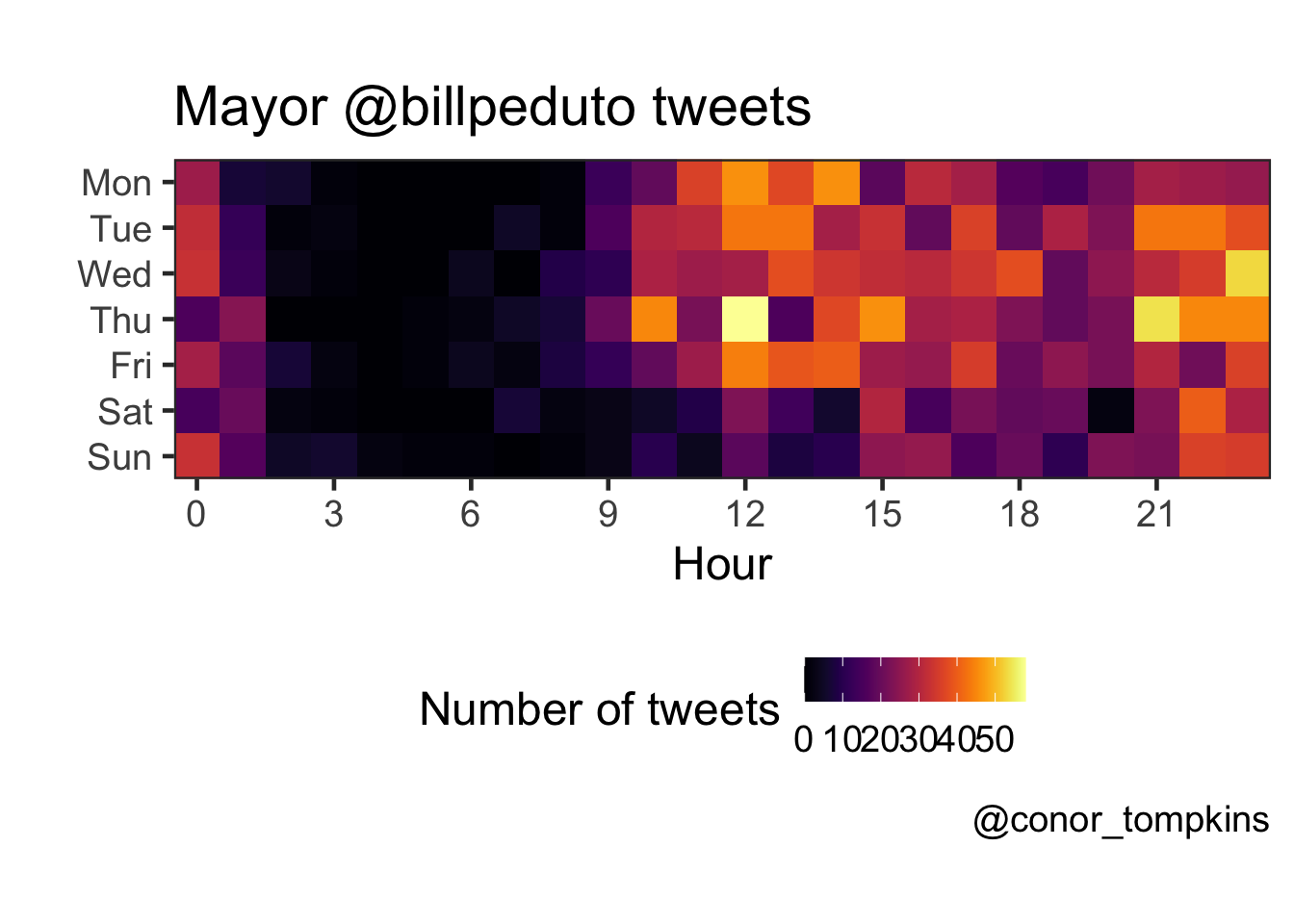This plot shows the percentage of the Mayor’s tweets that are regular tweets, retweets, quotes, and replies.

``````df_bill %>%
select(date, month_year, month, week, is_retweet, is_quote, reply_to_screen_name) %>%
is_retweet == FALSE & is_quote == FALSE ~ "Regular tweet",
is_retweet == TRUE ~ "Retweet",
is_quote == TRUE ~ "Quote")) -> df_bill_tweet_types

df_bill_tweet_types %>%
count(month_year, tweet_type) %>%
mutate(tweet_type = factor(tweet_type, levels = rev(c("Retweet", "Regular tweet", "Quote", "Reply")))) -> df_bill_tweet_types_month_year

df_bill_tweet_types_month_year %>%
ggplot(aes(month_year, n, fill = tweet_type, group = tweet_type)) +
geom_area(position = "fill") +
scale_fill_viridis(name = "Tweet type", discrete = TRUE) +
scale_x_discrete(expand = c(0,0)) +
scale_y_continuous(expand = c(0,0), labels = scales::percent) +
labs(title = "Types of @billpeduto tweets",
x = "",
y = "Percentage of tweets",
caption = caption)``````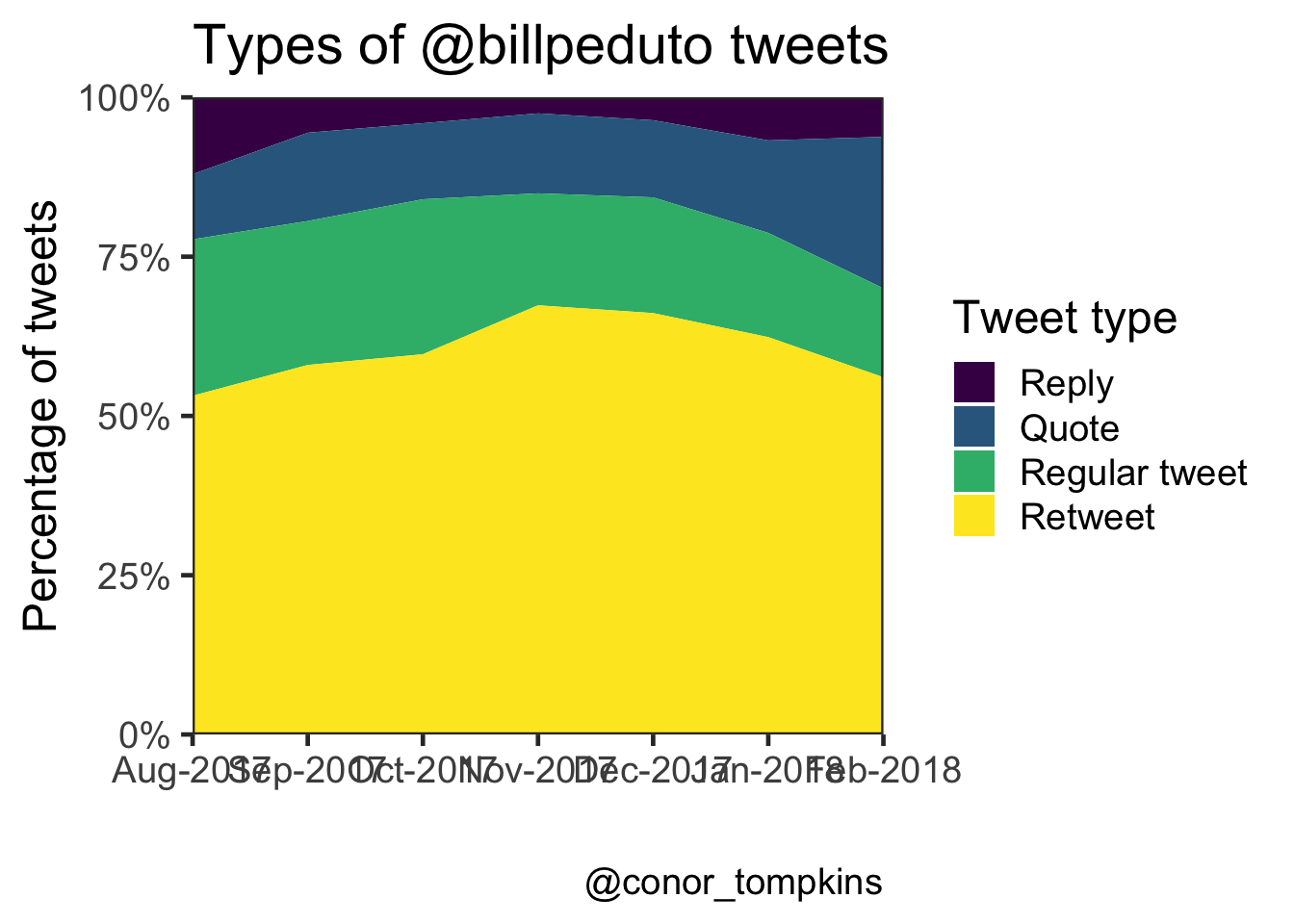This heatmap shows that the Mayor replies to tweets most often in the late evening.

``````df_bill %>%
filter(!is.na(reply_to_screen_name), is_quote == FALSE, is_retweet == FALSE) %>%
count(wday, hour) %>%
complete(wday, hour = 0:23) %>%
replace_na(list(n = 0)) %>%
ggplot(aes(hour, wday, fill = n)) +
geom_tile() +
coord_equal() +
scale_x_continuous(expand = c(0,0),
breaks = seq(0, 24, by = 3)) +
scale_y_discrete(expand = c(0,0)) +
scale_fill_viridis(option = 3) +
labs(x = "Hour",
y = "Day of the week") +
guides(fill = guide_colorbar("Number of tweets")) +
theme(legend.position = "bottom")``````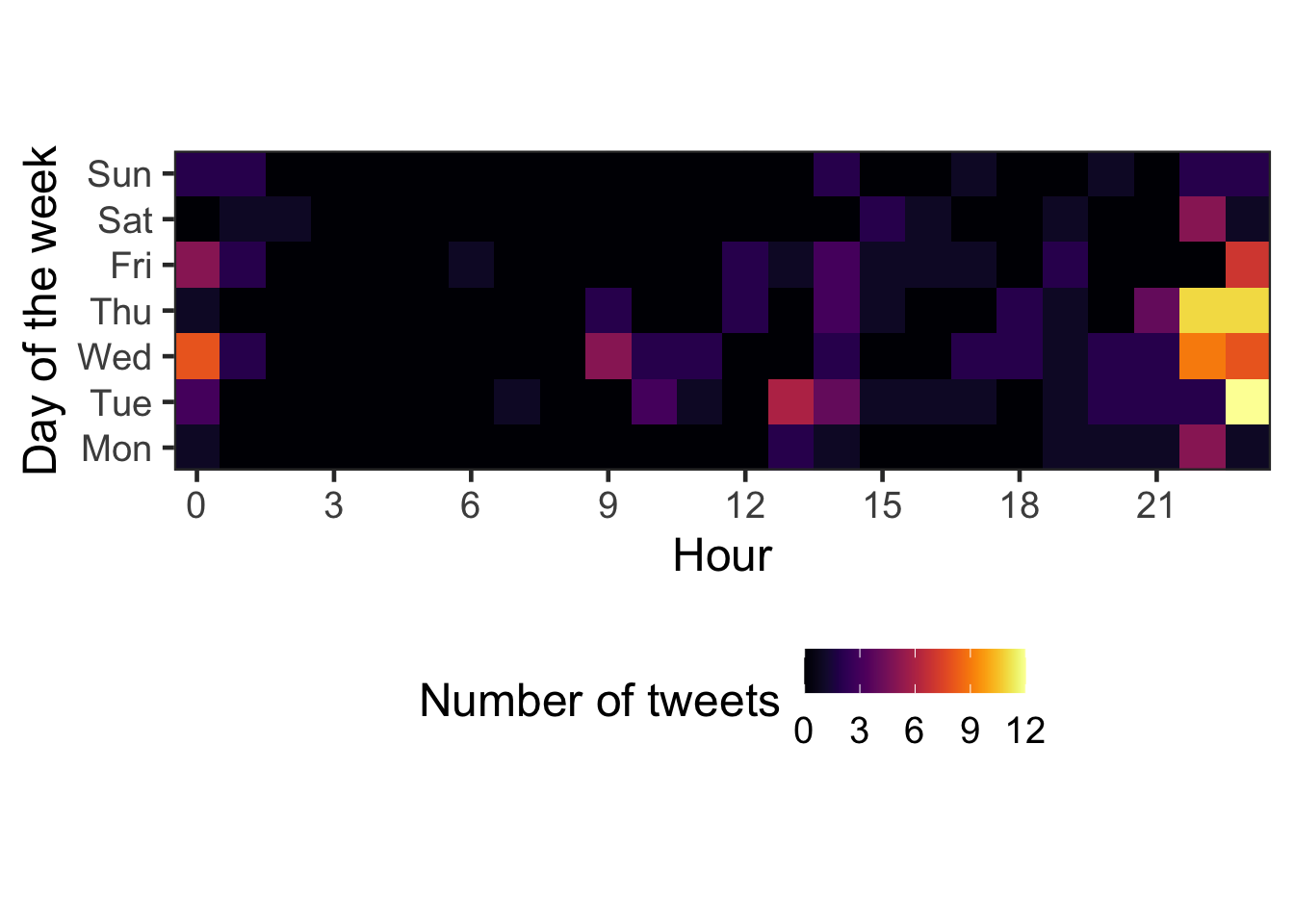## Text Analysis

The R package Tidytext makes it very easy to parse text data and pull insights from it. It interfaces very easily with ggraph, which creates network graphs.

``````set.seed(1234)

By separating the Mayor’s tweets into bigrams (two-word chunks), we can identify which words are used together. This code cuts the tweets into bigrams and counts their occurences. I exclude retweets and quotes from this analysis.

``````count_twitter_bigrams <- function(dataset, custom_stopwords) {
replace_reg <- "https://t.co/[A-Za-z\\d]+|http://[A-Za-z\\d]+|&amp;|&lt;|&gt;|RT|https|'s"

dataset %>%
filter(is_quote == FALSE, is_retweet == FALSE) %>%
mutate(text = str_replace_all(text, replace_reg, "")) %>%
unnest_tokens(bigram, text, token = "ngrams", n = 2) %>%
separate(bigram, c("word1", "word2"), sep = " ") %>%
filter(!word1 %in% stop_words\$word,
!word2 %in% stop_words\$word,
!word1 %in% custom_stopwords,
!word2 %in% custom_stopwords) %>%
count(word1, word2, sort = TRUE)
}

bill_stop_words <- c("t.co", "https", "amp")

tweets_bill %>%
rename(count = n) %>%
kable("html") %>%
kable_styling()``````
word1 word2 count
public safety 12
affordable housing 10
amazon hq2 8
climate change 6
common sense 6
u.s cities 6

This network graph shows which words the Mayor uses together frequently. “public” + “safety”, and “affordable” + “housing” are the main standouts. I think this plot pretty accurately captures the topics the Mayor tweets about.

``````visualize_bigrams <- function(bigrams, minimum, text_size = 3, title = NULL, subtitle = NULL, caption = NULL) {
set.seed(2016)
a <- grid::arrow(type = "closed",
length = unit(.1, "inches"))

bigrams %>%
filter(n >= minimum) %>%
graph_from_data_frame() %>%
ggraph(layout = "fr") +
geom_node_point(color = "lightblue", size = 3) +
geom_node_text(aes(label = name), size = text_size, vjust = 1, hjust = 1) +
geom_edge_link(aes(edge_alpha = n, edge_width = n), show.legend = TRUE, arrow = a, end_cap = circle(.25, 'inches')) +
scale_edge_width_continuous("Count", range = c(.5, 1.5)) +
scale_edge_alpha_continuous("Count", range = c(.3, .7)) +
labs(title = title,
subtitle = subtitle,
caption = caption) +
theme_void(base_size = 18)
}

visualize_bigrams(tweets_bill,
minimum = 4,
text_size = 5,
title = "@billpeduto tweets",
subtitle = "Bigram network",
caption = caption)``````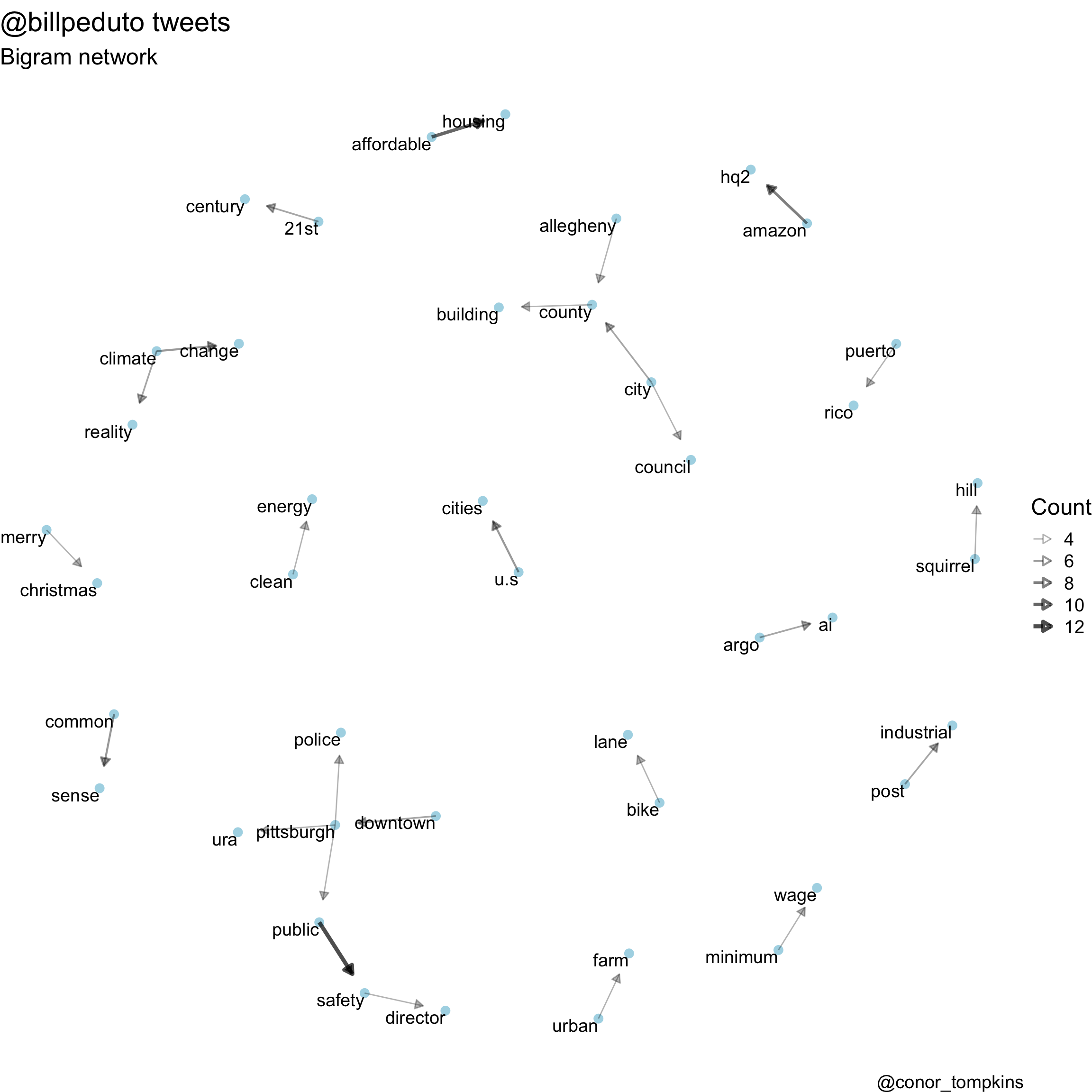This code determines which words are correlated with each other:

``````word_correlations <- function(dataframe, minimum, custom_stopwords){
replace_reg <- "https://t.co/[A-Za-z\\d]+|http://[A-Za-z\\d]+|&amp;|&lt;|&gt;|RT|https"
unnest_reg <- "([^A-Za-z_\\d#@']|'(?![A-Za-z_\\d#@]))"
dataframe %>%
filter(is_quote == FALSE, is_retweet == FALSE) %>%
select(status_id, text) %>%
mutate(section = row_number() %/% 10) %>%
filter(section > 0) %>%
mutate(text = str_replace_all(text, replace_reg, "")) %>%
unnest_tokens(word, text, token = "regex", pattern = unnest_reg) %>%
filter(!word %in% stop_words\$word,
!word %in% custom_stopwords,
str_detect(word, "[a-z]")) %>%
mutate(word = str_replace(word, "'", ""),
word = str_replace(word, "'", ""),
word = SnowballC::wordStem(word)) %>%
group_by(word) %>%
filter(n() >= minimum) %>%
pairwise_cor(word, section, sort = TRUE)
}

bill_stopwords <- c("t.co", "https", "amp")

bill_words <- word_correlations(df_bill, minimum = 10, custom_stopwords = bill_stopwords)``````

This plot shows which words are correlated highly with each other. Some words are “stemmed” (shortened and/or modified), to capture very similar words. For example, “business” and “businesses” could be stemmed to “busine”.

“amazon” and “hq2” are used together overwhelmingly. The “pwsa” + “water” + “decad” combination indicate the ongoing water quality problems, and the plans to reinvest in the infrastructure. This plot shows how some of the policy issues the Mayor focuses on are connected.

``````visualize_word_correlations <- function(dataframe, title, subtitle, caption){
dataframe %>%
filter(correlation > .3) %>%
graph_from_data_frame() %>%
ggraph(layout = "fr") +
geom_edge_link(aes(edge_alpha = correlation, edge_width = correlation), show.legend = FALSE) +
geom_node_point(color = "lightblue", size = 5) +
geom_node_label(aes(label = name), size = 5, repel = TRUE) +
scale_edge_alpha_continuous(range = c(.1, .5)) +
theme_void(base_size = 18) +
labs(title = title,
subtitle = subtitle,
caption = caption)
}

visualize_word_correlations(bill_words,
title = "@billpeduto tweets",
subtitle = "Word correlation network",
caption = "@conor_tompkins")``````